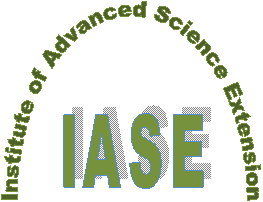IJAAS International journal of ADVANCED AND APPLIED SCIENCES EISSN: 2313-3724, Print ISSN:2313-626X Frequency: 12Volume 4, Issue 12 (December 2017), Pages: 133-144----------------------------------------------  Original Research Paper  Title: A new approach for solving compressible Navier-Stokes equations  Author(s): A. S. J. Al-Saif *, Takia Ahmed J. Al-Griffi  Affiliation(s):  Department of Mathematics, College of Education for Pure Sciences, Basrah University, Basrah, Iraq  Abstract: In this paper, we introduce the analytical approximate solutions for one and two-dimension compressible Navier-Stokes equations by applying a relatively new method named splitting decomposition homotopy perturbation method. The new methodology depends on combining Adomian decomposition and Homotopy perturbation methods with the splitting time scheme for differential operators. The numerical results which we obtained from the solutions of the two problems, show that the new method is efficient with good converge and high accuracy compared with the two standard methods i.e. Adomian decomposition method and Homotopy perturbation method.   © 2017 The Authors. Published by IASE.  This is an open access article under the CC BY-NC-ND license (http://creativecommons.org/licenses/by-nc-nd/4.0/).  Keywords: Splitting scheme, Adomian decomposition, Homotopy perturbation method, Compressible, Navier-Stokes equation, Convergence analysis  Article History: Received 13 July 2017, Received in revised form 12 October 2017, Accepted 12 October 2017  Digital Object Identifier:   Citation:  Al-Saif ASJ and Al-Griffi TAJ (2017). A new approach for solving compressible Navier-Stokes equations. International Journal of Advanced and Applied Sciences, 4(12): 133-144  Permanent Link: ----------------------------------------------  References (25) Adomian G (1988). A review of the decomposition method in applied mathematics. Journal of Mathematical Analysis and Applications, 135(2): 501-544. https://doi.org/10.1016/0022-247X(88)90170-9  Ali AH and Al-Saif ASJ (2008). Adomian decomposition method for solving some models of nonlinear Partial Differential Equations. Journal of Basrah for Sciences, 26: 1-11.      Alkalla ILH, Abd-Elmonem RA, and Gomaa AMAA (2013). Convergence of discrete adomian method for solving a class of nonlinear fredholm integral equations. Applied Mathematics, 4(01): 217-222. https://doi.org/10.4236/am.2013.41A033  Al-Saif ASJ (2015). Analytical approximate solutions for two-dimensional incompressible Navier-Stokes equations. Advances in Physics Theories and Application, 49: 69-86.      Al-Saif ASJ and Al-Griffi J (2017). Splitting decomposition homotopy perturbation method to solve one-dimensional NSE. Journal of Advances in Mathematics, 13(2):7123-7134.      Biazar J and Aminikhah H (2009). Study of convergence of homotopy perturbation method for systems of partial differential equations. Computers and Mathematics with Applications, 58(11): 2221-2230. https://doi.org/10.1016/j.camwa.2009.03.030  Çelik E, Bayram M, and Yeloglu T (2006). Solution of differential-algebraic equations (DAEs) by adomian decomposition method. International Journal of Pure and Applied Mathematics, 3(1): 93-100.      Fonseca F (2016). Solutions of one and two-dimensional compressible Navier-Stokes equations. Advanced Studies in Theoretical Physics, 10(3): 145-155. https://doi.org/10.12988/astp.2016.613  Ganji DD, Afrouzi GA, Hosseinzadeh H, and Talarposhti RA (2007). Application of homotopy-perturbation method to the second kind of nonlinear integral equations. Physics Letters A, 371(1): 20-25. https://doi.org/10.1016/j.physleta.2007.06.003  He JH (1999). Homotopy perturbation technique. Computer Methods in Applied Mechanics and Engineering, 178(3): 257-262. https://doi.org/10.1016/S0045-7825(99)00018-3  Hemeda AA (2012). Homotopy perturbation method for solving systems of nonlinear coupled equations. Applied Mathematical Sciences, 6(93-96): 4787-4800.      Inc M (2004). On numerical solution of partial differential equation by Adomian decomposition method. Kragujevac Journal of Mathematics, 26: 153-164.      Inc M (2005). On numerical solutions of one-dimensional nonlinear Burgers' equation and convergence of the decomposition method. Applied Mathematics and Computation, 170(1): 76-85. https://doi.org/10.1016/j.amc.2004.10.069  Jang B (2007). Solutions to the non-homogeneous parabolic problems by the extended HADM. Applied Mathematics and Computation, 191(2): 466-483. https://doi.org/10.1016/j.amc.2007.02.132  Jin L (2008). Homotopy perturbation method for solving partial differential equations with variable coefficients. International Journal of Contemporary Mathematical Sciences, 3(28): 1395-1407.      Liao SJ (1995). An approximate solution technique not depending on small parameters: A special example. International Journal of Non-Linear Mechanics, 30(3): 371-380. https://doi.org/10.1016/0020-7462(94)00054-E  Liao SJ (1997). Boundary element method for general nonlinear differential operators. Engineering Analysis with Boundary Elements, 20(2): 91-99. https://doi.org/10.1016/S0955-7997(97)00043-X  Luo XG (2005). A two-step Adomian decomposition method. Applied Mathematics and Computation, 170(1): 570-583. https://doi.org/10.1016/j.amc.2004.12.010  Luo XG, Wu QB, and Zhang BQ (2006). Revisit on partial solutions in the Adomian decomposition method: solving heat and wave equations. Journal of Mathematical Analysis and Applications, 321(1): 353-363. https://doi.org/10.1016/j.jmaa.2005.08.043  Perron S, Boivin S, and He'rad J (2004). A new finite volume method to solve three-dimensional Navier-Stokes equation on unstructured meshes. Computers and Fluid, Elsevier, 33(10):1305-1333. https://doi.org/10.1016/j.compfluid.2003.10.006  Seng V, Abbaoui K, and Cherruault Y (1996). Adomian's polynomials for nonlinear operators. Mathematical and Computer Modelling, 24(1): 59-65. https://doi.org/10.1016/0895-7177(96)00080-5  Shahmohamadi H and Mohammadpour M (2014). A series solution for three-dimensional Navier-Stokes equations of flow near an infinite rotating disk. World Journal of Mechanics, 4(5):117-127. https://doi.org/10.4236/wjm.2014.45014  Wahab H, Jamal A, Bhatti S, Naeem M, Shahzad M, and Hussain S (2015). Application of homotopy perturbation method to the Navier-Stokes equations in cylindrical coordinates. Computational Ecology and Software, 5(2): 139-151.      Zhang B and Lu J (2011). Exact solutions of homogeneous partial differential equation by a new adomian decomposition method. Procedia Environmental Sciences, 11: 440-446. https://doi.org/10.1016/j.proenv.2011.12.070  Zhu Y, Chang Q, and Wu S (2005). A new algorithm for calculating Adomian polynomials. Applied Mathematics and Computation, 169(1): 402-416. https://doi.org/10.1016/j.amc.2004.09.082